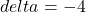# On Average Jun 2022

Here’s the whole story visually:The rest is details.

Most everyone in math knows these tricks — here’s a summary of techniques used to make quick mental calculations of averages.

In the problems below, consider the residual,

R is defined as the remainder above or below the guessed average, aka, the residual.

RS is defined as the residual sum.

1. In school, I have 8 exam scores; what’s my average?
90
92
88
95
97
81
85
100
~~~STEP by STEP (with explanation)
Guess maybe 90, then mentally calculate the running residual sum using two or three numbers at a time:
say zero (for 90: 90 is neither above or below the guessed average ∴R=0; ∴RS = 0),
say zero (for 92 and 88: the average of 92 and 88 is neither above or below the guessed average ∴R=0; ∴RS = 0),
say 3 (for 95, 97, and 81: ∴R = 5 + 7 – 9; ∴RS = 3),
say 8 (for 85 and 100: ∴R= -5 + 10 = 5; ∴RS = 8).
Answer: The average is 90 + 8/8 = 91.

2. Same problem — different guess. In school, I have 8 exam scores; what’s my average?
90
92
88
95
97
81
85
100
~~~STEP by STEP (with explanation)
Guess maybe 85, then mentally calculate the running residual sum using two or three numbers at a time:
say 15 (for 90, 92, 88: ∴R = 5 + 7 + 3 = 15; ∴RS = 15),
say 37 (for 95 and 97: ∴R = 10 + 12 = 22; ∴RS = 37),
say 48 (for 81, 85, and 100: ∴R = -4 + 0 + 15 = 11; ∴RS = 48),
Answer: The average is 85 + 48/8 = 91.

3. Find average of 5 ‘hard numbers’ (with a lot of 9s, 8s, and 7s):
987
965
882
949
767
~~~STEP by STEP (with explanation)
Assume a basis of 1000, mentally calculate the running residual sum:
say 13 (for 987: ∴R = -13; ∴RS = 13 down),
say 48 (for 965: ∴R = -35; ∴RS = 48 down),
say 99 (for 949: ∴R = -51; ∴RS = 99 down),
say 217 (for 882: ∴R = -118; ∴RS = 217 down),
say 450 (for 767: ∴R = -233; ∴RS = 450 down),
Answer: The average is 1000 – 450/5 = 910.

4. Incrementing an average, for example, I have 8 exam scores:
90
92
88
95
97
81
85
100
with an average of 91 (see Problem 1). Now I have a score of 78; what is my new average without re-summing?
Answer: It is simply 91 + (78 – 91)/9 = 91 – 13/9 = 89 5/9 = 89.55
Formula:5. [Powerful Formula] Incrementing an average, for example, I have 100 exam scores with an average of 87. Now I have a score of 100; what is my new average without re-summing?
Answer: It is simply 87 + (100 – 87)/101 = 87.1287

6. Incrementing an average, for example, I have 10,000 exam scores with an average of 87. Now I have a score of 100; what is my new average without re-summing?
Answer: It is simply 87 + (100 – 87)/10001 = 87.0013

7. [Very Powerful Formula] Incrementing an average, for example, I have 100 exam scores with an average of 87. Now I have 3 scores of {85, 80, 75}; what is my new average without re-summing?
87 – 85 = 2
87 – 80 = 7
87 – 75 = 12
Answer: It is simply 87 – (2 + 7 + 12)/103 = 86.7961

8. Determine the lowest allowable score to maintain a certain average. Say I have 8 scores with an average of 94. What is the least score on my 9th exam to maintain at least an average of 90?
Answer: It is 94 + (90 – 94) · 9 = 58.
Formula:9. [Very Very Powerful Formula] Determine the lowest allowable final exam score to maintain a certain average. Say I have 8 scores with an average of 94. What is the least score on my final exam to maintain at least a course grade of 90? Assume that the final exam is 40% of my course grade.
Answer: It is [90 – (94 x 0.6)]/0.4 = 84.
Formula:[Wicked Powerful Formula] Alternative and easier formula:10. So, how much does a 100 raise your average?
Formula:Enjoy!